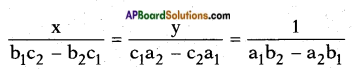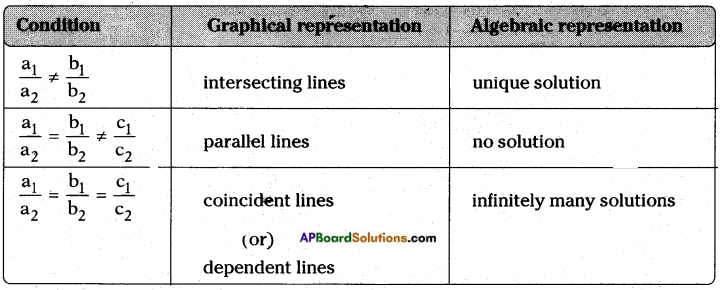# AP SSC 10th Class Maths Notes Chapter 4 Pair of Linear Equations in Two Variables

Students can go through AP SSC 10th Class Maths Notes Chapter 4 Pair of Linear Equations in Two Variables to understand and remember the concepts easily.

## AP State Syllabus SSC 10th Class Maths Notes Chapter 4 Pair of Linear Equations in Two Variables

→ An equation of the form ax + by + c = 0 where a, b, c are real numbers and where at least one of a or b is not zero (i.e, a2 + b2 ≠ 0) is called a linear equation in two variables x and y.

→ A pair of equations in the same two variables forms a pair of linear equations. The system of pair of equations in general a1x + b1y + c1 = 0 ; a2x + b2y+ c2 = 0
where a1, a2, b1, b2, c1, c2 ∈ R such that a12 + b12 ≠ 0 and a22 + b22 ≠ 0→ A pair of linear equations in two variables can be represented and solved by
a) Graphical method
b) Model method
c) Algebraic methods
i) Substitution method
ii) Elimination method
iii) Cross-multiplication method.

→ Graphical method: The two linear equations in two variables are represented by two straight lines on a graph sheet.
a) If they intersect at a point, then the point gives the unique solution of the two equations. In this case the two equations are consistent.
b) If the lines coincide, then there are infinitely many solutions. Each point on the line represents a solution. In this case, we say that the pair of equations is dependent or consistent.
c) If the two lines are parallel to one another, then the pair of equations has no solution. In this case we say that the pair of equations is inconsistent. Substitution method : In this method, we make one of the variables x or y as the subject from the first equatiori. We substitute this value in the second equation and get the value of the variable involved, then by substituting this value in any of the equations we get the value of second variable.
Eg : 2x + 4y = 16 …….. (1)
3x – 8y = – 18 …….. (2)
From equation (1); 2x + 4y = 16 ⇒ 2x = 16 – 4y ⇒ x = $$\frac{16-4y}{2}$$
Substituting x = $$\frac{16-4y}{2}$$ in the second equation
$$3\left(\frac{16-4 y}{2}\right)$$ – 8y = -18
48 – 12y – 16y = – 18 × 2
-28y = -36 – 48
y = $$\frac{-84}{-28}$$ = 3
Substituting y = 3 in (1), 2x + 4y = 16
2x + 4(3) = 16
2x = 16 – 12
x = 2→ Elimination method: In this method we first try to eliminate one of the two variables and by reducing the system to equation in one variable. We then solve for the variable.
The following steps may be adopted:
Step – 1: Multiply both the equations by some suitable non-zero constants to make the co-efficients of one variable (either x or y) numerically equal.
Step – 2: Add or subtract one equation from other so that one variable gets eliminated. If we get an equation in one variable, proceed to step 3.
If in step – 2, we obtain a true statement involving no variable, then the original pair of equations has infinitely many solutions.
If in step – 2, we obtain a false statement involving no variable, then the original pair of equations has no solutions, i.e., it is inconsistent.
Step – 3: Solve the equation in one variable (x or y) so obtained to get its value.

→ Cross-multiplication method: Let the pair of equations be a1x + b1y + c1 = 0 ; a2x + b2y+ c2 = 0→ In every case, the obtained solutions should always be verified with the original equations.
Let a1x + b1y + c1 = 0 and a2x + b2y+ c2 = 0, form a pair of linear equations. Then the following situations can arise,
Case – (i): $$\frac{a_{1}}{a_{2}}$$ ≠ $$\frac{b_{1}}{b_{2}}$$ – pair of linear equations is consistent.
Case – (ii): $$\frac{a_{1}}{a_{2}}$$ = $$\frac{b_{1}}{b_{2}}$$ ≠ $$\frac{c_{1}}{c_{2}}$$ – pair of linear equations is inconsistent.
Case – (iii): $$\frac{a_{1}}{a_{2}}$$ = $$\frac{b_{1}}{b_{2}}$$ = $$\frac{c_{1}}{c_{2}}$$ – pair of linear equations is dependent and consistent.→ There are several situations which can be mathematically represented by two equa¬tions that are not linear to start with. But we alter them so that they are reduced to a pair of linear equations.

→ The pair of values of the variables x and y for which the pair of equations is satisfied is called the solution pair.